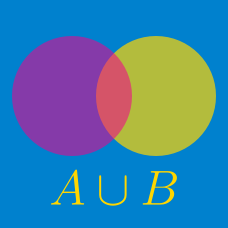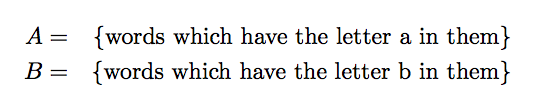Probability

# Sets - Composition of OperationsWhat is

$( A \backslash B ) \cup ( B \backslash A)?$

If $A=\{2,5,6,18,20\}, B=\{1,2,6,20,22\},$ then which of the following is equal to $\left((A \setminus B) \cup (B \setminus A)\right) \cap A?$

Details and assumptions

You may choose to read the summary page Set Notation.

Consider the ground set $U=\{x \mid x \mbox{ is a non-negative integer } \leq 9\}$ and let $A$ and $B$ be two subsets of $U.$ If $A^c \cap B^c=\{8, 9\},$ what is $\lvert A \cup B \rvert?$

The sets $A=\{12,14,16\}, B=\{13,15,17\}$ are subsets of a universal set $U$. If $A \cup B=U,$ then what is the sum of the elements of the set $\left((A \cap B) \cup (A^{C} \cap B)\right) \cup \left((A^{C} \cap B^{C}) \cup (A \cap B^{C})\right)?$

Details and assumptions

You may choose to read the summary page Set Notation.

Suppose $A$ and $B$ are the following subsets of a universal set $U$: $A=\{1,3,5,10,13,23\}, B=\{2,4,6,14\}.$ What is the number of elements of the set $A \setminus \left((A \setminus B) \cup \left(A \setminus B^{C}\right)\right)?$

Details and assumptions

You may choose to read the summary page Set Notation.

×